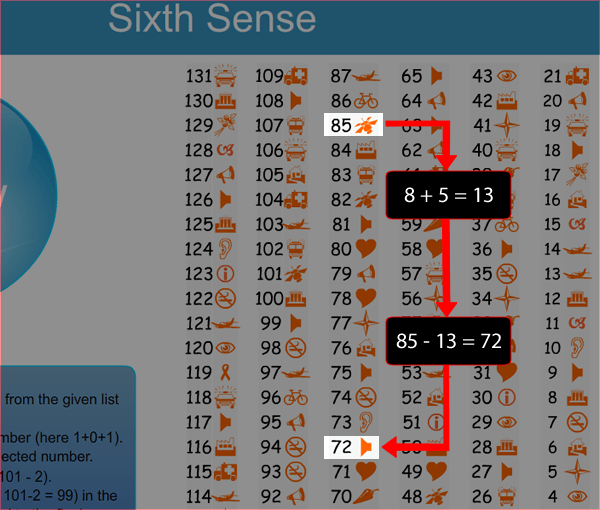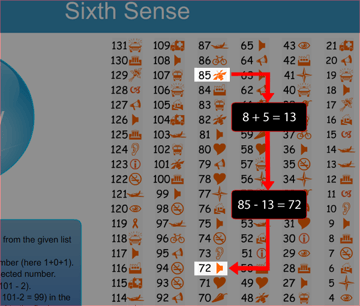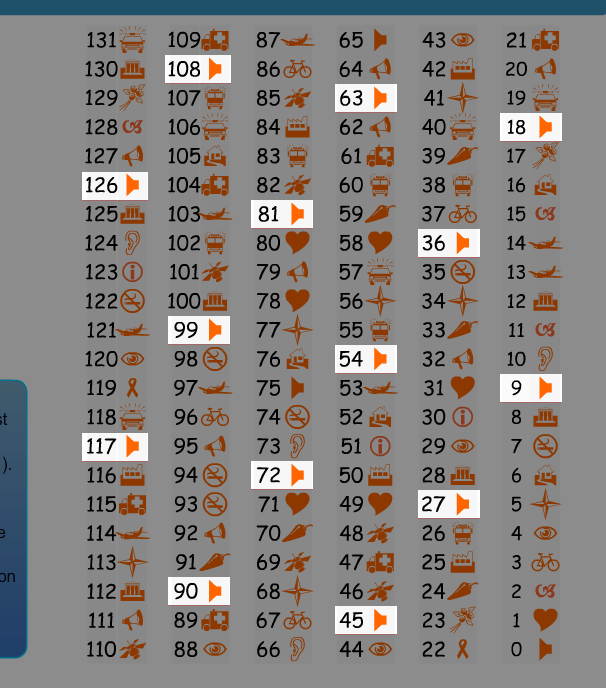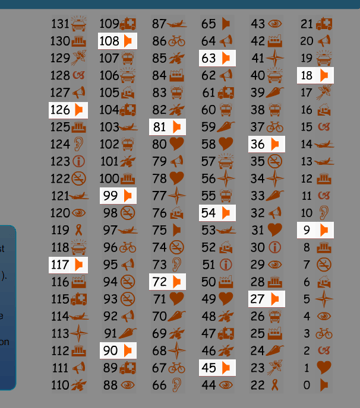# Become a Flash Mathemagician With Fun Math Tricks

Good to see you here on Activetuts+. You want to be a "Mathemagician"? All you need is some simple math from your notebook! What? You're no good at math? Don't worry. Neither am I. So I looked back into my school days and collected some math tricks we often used for fun. In this article we shall see some of those tricks and how to use them to build interesting fun applications.

First let us have a look at the following fun application I created in Flash. Actually, I was inspired to build this app when I saw a similar app while surfing the net.

This fun application "Sixth Sense" is a good example of "How to use maths tricks in real life applications."

## Sixth Sense: Fun Flash Application

We'll discuss this math trick used in this fun application later in this session.

Read the instructions before you click Show!

## Exploring the Math Trick Behind the Scene

The trick behind this application is so easy that after knowing it you will experience "Brain quake", of above 8 on Richter scale, for sure.

To understand this trick you just have to revise the nine times table you studied in your school days. This was the easiest table to learn, I thought.

If you concentrate on the final answer in the SWF you will find that the number will always be a multiple of 9.

For e.g. If you choose 85, the final number after doing those tricky maths steps will be 72. Aaha... 72 is multiple of 9 (i.e. 9 x 8 = 72).Try another one. Consider number 86. So, 8 + 6 = 14. Then, 86 - 14 = 72. Haha... 72 again.

Try another one. Consider number 40. So, 4 + 0 = 4. Then, 40 - 4 = 36. And of course 36 is multiple of 9 (i.e. 9 x 4 = 36).

Now do you have some idea of how things work?. Along with the nine times table you must also consider those math steps which always give a multiple of nine.

So we will take a look at those steps. First, the sum of the digits of the selected number is done (For e.g. 105 will be 1 + 0 + 5 = 6).

Then the resulting number is subtracted from the selected number (i.e. 105 - 6 = 99).

Initially I tried it in a slightly different way (e.g. If 85, then, 8 + 5 = 13. Then, 1 + 3 = 4. Finally, 85 - 4 = 81. Thus we have multiple of 9 i.e. 81). But the previous example is more effective, as it is simpler.

And last but not least, about "Symbols". Why symbols? There are symbols against each number. So that user can compare it with his final answer.

These symbols here are placed cleverly to create the feel of magic. Want to know how? Observe every number which is a multiple of nine and check their symbols. Surprised? They all are same and will always be same. This is the crux of the whole process. That's why they are programmed to do so.Very smart. First you are told to pick up a number. Then doing some tricky maths with this number you are brought to a resulting number which is multiple of nine. As you know for all these multiple of nine numbers same symbol is already set. Other symbols are mixed up into this reserved symbol to hide the trick.

The "Show" button will always show this reserved symbol. Thus the user gets shocked and starts wondering how it worked.

Congrats. Now you know how things can be made magical using the power of maths and programming.

[Editor's Note: So how many of you can explain why this works? I've figured out a proof, honest, but sadly this sidebar is too small to contain it. If you've got an explanation as well, stick a note in the comments.]

## More Maths Tricks

This was one application and one math trick. How about some other math tricks and possible fun applications?

I have collected some math tricks we often used in school days. I think these tricks might be useful to build some similar fun applications.

• Trick 1: Number Guess
• Trick 2: Guess missing number (Yet another trick using nine times table)
• Trick 3: Guess Birth Date
• Trick 4: Guess the perfect number
• Trick 5: Guess the age

We shall discuss these tricks, how they works and the possibilities of using them for creating fun applications in the rest of this article.

## Trick 1: Number Guess

This is a very interesting trick. We used this trick so many times in my school days that I still remember it. Let's take a look.

1. Ask the user to think of any number silently in his mind (suppose it is 50).
2. Tell him his friend has the same number, so he should add them together. (Now it is 50 + 50 = 100)
3. Give him your number (Say 150) and tell him to add it in the total (100 + 150 = 250)
4. Tell him to divide the total by 2 (250/2 = 125)
5. Tell him to give his friend's number back to him. (125 - 50 = 75). Tell him to remember this total in his mind.
6. Now, if you divide your number (i.e. 150) by 2, you'll get his final number (e.g. 150/2 = 75). Simply announce your answer which is equal to user's answer and watch the shocked look on his face.

The key part of this trick is "your number" and "dividing" it by ,"2". Thus regardless of the user number the answer is always with you.

So whatever the user thinks, the final result will always be the half of "your number". For e.g. If you give 100 to add to the total, then the final answer will be 100/2 = 50.

How to use this trick to build an interesting fun application?

Take a look at the following fun application i created in Flash. It uses the above "Number Guess" trick.

Yet another excellent example of putting math tricks in the playground.

## Trick 2: Guess the Missing Number

This is yet another trick using nine times table.

Tell the user to get pencil and paper (this is needed since we are going to tell him to cross out any number from final answer). And one more thing; this trick is best suited to a four digit number.

1. Ask him to write down any number (At least four digits long). e.g. 6534.
2. Ask him to sum up the digits e.g. 6+5+3+4 = 18.
3. Tell him to subtract the above resulting number (i.e. 18) from the original number (i.e.6534) as, 6534 - 18 = 6516.
4. Tell the user to cross out any number (except zero (0) if any) from the above answer (Say, he crossed out 5, to get 6X16)
5. Tell him to type the remaining digits (excluding the crossed out number, so like 616) in a text box in your application.

Once the remaining number is with you then guessing the missing number is simply a case of applying some tricky math steps.

Your application will sum up the digits typed by the user (as 6+1+6 = 13). Now with this number 13 all you have to do is tell your application to find out next nearest multiple of nine. In this case, 18 is the next nearest multiple of nine number after 13.

Now you are just one step away to find out missing number, the one that was crossed out. Tell your application to subtract the multiple of nine from the summed digits... so, 18 - 13 = 5 and this 5 is nothing but the crossed out number. Magic...Isn't it?

Display this number with some nice animation creating the feel of magic.

## Trick 3: Guess Birth Date

Not such a great trick, but building a fun application with this trick is still possible.

1. Tell the user to get some paper, a pencil, and a calculator.
2. On the paper tell him to add 18 to his birth month.
3. Multiply by 25.
4. Subtract 333.
5. Multiply by 8.
6. Subtract 554.
7. Divide by 2.
8. Tell the user to add his birth date.
9. Multiply by 5.
11. Multiply by 20.
12. Tell him to add last two digits of user's birth year.
13. Subtract 32940.

If the answer is 32089 then the birth date is March 20th, 1989. (3/20/89)

To put this in application form you may collect the final answer as an input from the user in your application. Then program to convert this answer into mm/dd/yy format

Finally display this birth date with nice animation.

You can also guess the age with this data if user's computer date is correct.

## Trick 4: Guess the Exact Number

Unfortunately, this trick requires a four-digit number. Let's take a look.

1. Tell the user to write any four-digit number (say, 5623).
2. Tell him to write down first digit (here, 5).
3. Now write first two digits (here, 56).
4. And lastly first three digits (here, 562).
5. Now sum up above three numbers from three steps (as, 5 + 56 + 562 = 623).
6. Multiply the answer by 9 (as, 623 x 9 = 5607).
7. Sum up the 4 digits of the original number (i.e. 5623) of step1 (as, 5 + 6 + 2 + 3 = 16).
8. Now add these last two results (as, 5607 + 16 = 5623). Boom..... this is the original number.

I know what you are thinking: 'How do I apply this trick to a fun game?'. Well let's see..

Your application could instruct the user to provide results from Steps 6 and 7 as inputs.

With these inputs your program would give the final number. Display this number on the screen to amaze the user.

## Trick 5: Guess the Age

And one more trick with the number 9 (this really is a mysterious number). Play with it and you will discover many tricks with it. (Note: this trick is best suited for those aged between 10 to 100.)

1. Tell the user to think or write his age on the paper (e.g. age is 35).
2. Then tell him to add 90 to his age (i.e. 35 + 90 = 125).
3. Tell him to write the last two digits of the above answer (here, 25).
4. Tell him to add 1 to the above number (as, 25 + 1 = 26).
5. If you add 9 to the above answer you will get the age of the user (here, 26 + 9 = 35).

To put this in application form get the answer from the second-last step (here, 26) as the input and simply add 9 using the programming logic. Display this final answer as the user's age.

That's it for now.

## Conclusion.

So friends, feeling inspired enough to create fun applications using the power of math? I am also eager to build some more apps.

Math tricks and fun applications. Possibilities are endless. It's a matter of picking up a perfect math trick.

Good luck!